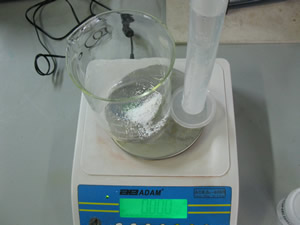Determination of stoichiometric ratio.

5.00g Calcium carbonate powder
Spatula
200 mL beaker
100 mL measuring cylinder
Electronic scales
2 M HCl solution
Goggles, glove and lab-coate

Acids react with carbonates to produce carbon dioxide, water and a salt.

Calcium carbonate will react with hydrochloric acid to produce carbon dioxide, water and calcium chloride according to the equation below.

CaCO3(s) + 2HCl(aq) => H2O(l) + CO2(g) + CaCl2(aq)

According to the equation, one mole of calcium carbonate will produce one mole of carbon dioxide gas. The stoichiometric ratio is 1:1 The aim of this experiment is to verify that the ratio really is 1:1.

1) Weigh approximately 5.00 grams of calcium carbonate on an electronic balance and place it into a 200 mL beaker.

2) Measure 40 mL of 2M HCl solution using a measuring cylinder.

3) Place the beaker containing the carbonate and the measuring cylinder with the acid onto the electronic balance as shown on the right. Press tare to zero.

4) Slowly add the acid to the carbonate. Take care not to add too much as the reactants will spill over onto the balance.

5) Add the acid until no more gas is observed to form.

6) Blow air on the beaker a few times and record the reading on the balance. You will notice a mass loss.

 Mass of calcium carbonate used (g) Mole of calcium carbonate Predicted mass of carbon dioxide formed Actual mass loss (g) Mole of carbon dioxide formed The calculated ratio between CaCO3 and CO2 formed. :What properties of carbon dioxide make step 6 necessary?